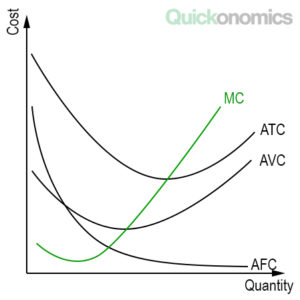# Three Properties of Cost Curves

| Updated Nov 29, 2019

Cost curves are a useful tool to analyze firm behavior. We can use a graph that shows average fixed cost (AFC), average variable cost (AVC), marginal cost (MC) and average total cost (ATC) to illustrate them (see also types of costs). Although the size and slope of the curves may vary, most of those graphs will look something like the one you can see below. In this graph, we can observe three important properties of cost curves that apply to most firms: (1) The marginal cost curve eventually rises as output increases, (2) the average total cost curve is U-shaped, and (3) the marginal cost curve intersects the average total curve at its bottom.## 1) Marginal Cost Curve Rises as Output Increases

As output increases, MC eventually rises. That means at some point in the production process it becomes more and more expensive to produce additional units of a good or service. This reflects the property of diminishing marginal productivity. When firms produce small quantities they often have idle resources that can be put to use, so the marginal product is high, while marginal costs (MC) are low. However, as the output increases, it becomes more difficult to add additional production capacity, which results in lower marginal returns and higher marginal costs.

To illustrate this, meet Roberto. Roberto owns a small barbershop, where he employs two barbers by the hour (i.e., as non-exempt employees). Because his team does such a great job, Roberto’s barbershop becomes increasingly popular and more and more customers visit his shop. As a result, his employees can serve more customers and work more hours. While the business grows further, Roberto decides to hire additional barbers. However, as the number of employees rises, the small barbershop becomes increasingly crowded. Roberto’s barbers sometimes need to wait to use the equipment and tread on each other’s toes. As a result, they can serve fewer customers per hour and their productivity decreases. In other words, due to the rising number of customers, the marginal cost of serving new clients rises, because the team needs more time and equipment to serve them.

Note that despite the increase in marginal costs described above, firms may experience falling marginal costs over a certain range of output. This usually happens at low levels of production when a lot of resources and capacity are idle and readily available.

## 2) Average Total Cost Curve is U-Shaped

For most firms, ATC is U-shaped. That means, as the output increases, the average total cost falls at first but eventually rises once production exceeds a certain level. Graphically speaking, this results in a U-shaped average total cost curve. The reason for this is that the average total cost curve reflects both average fixed costs (AFC) and average variable costs (AVC). On the one hand, AFC falls as output increases, because fixed costs can be spread over a larger number of units. On the other hand, AVC increase as the output rises, because of increasing marginal costs (see above). At low levels of production, the declining AFC usually outweighs the rising AVC, whereas the latter becomes more significant as the output grows.

For example, if Roberto only serves a small number of customers, his average total cost is high, because his fixed costs are only spread over a few clients. Meanwhile, as the number of customers increases, the average total costs decline until the growing number of clients causes his average variable costs to increase substantially.

Note that the bottom of the U-shape occurs at the quantity that minimizes the average total cost. Therefore, this quantity is sometimes also referred to as the efficient scale of the firm (i.e., because there is no quantity at which the firm could produce at lower average total costs).

## 3) Marginal and Average Total Cost Intersect at the Bottom of the Average Total Cost Curve

MC always intersects ATC at the minimum of the average total cost. That means, as long as the cost of producing an extra unit is lower than the average total cost (i.e., marginal cost < average total cost), ATC falls. Similarly, as soon as the cost of producing one more unit is higher than the current average total cost (i.e., marginal cost > average total cost), ATC rises. Therefore, the two curves intersect at the bottom of the average total cost curve, when MC equals ATC (i.e., marginal cost = average total cost).

In the case of our example, that means as long as Roberto’s marginal cost of serving an additional client is lower than the current average total cost, his ATC will fall. After all, an additional value that lies below the current average will always drag the average down. Likewise, as soon as the cost of serving another customer exceeds Roberto’s average total cost, his ATC curve will start to rise. Thus, the ideal number of customers for Roberto’s barbershop from a cost perspective is the quantity at which marginal cost equals average total cost.

## Summary

Cost curves are a useful tool to analyze firm behavior. In most cases, we can observe three properties of cost curves: (1) The marginal cost curve eventually rises as output increases, (2) the average total cost curve is U-shaped, and (3) the marginal cost curve intersects the average total curve at its bottom.